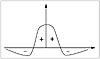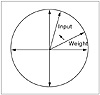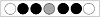# 76.C++ Neural Networks and Fuzzy Logic by Valluru B. Rao M&T Books, IDG Books Worldwide, Inc. ISBN: 1558515526   Pub Date: 06/01/95

## Chapter 11The Kohonen Self-Organizing Map

### Introduction

This chapter discusses one type of unsupervised competitive learning, the Kohonen feature map, or self-organizing map (SOM). As you recall, in unsupervised learning there are no expected outputs presented to a neural network, as in a supervised training algorithm such as backpropagation. Instead, a network, by its self-organizing properties, is able to infer relationships and learn more as more inputs are presented to it. One advantage to this scheme is that you can expect the system to change with changing conditions and inputs. The system constantly learns. The Kohonen SOM is a neural network system developed by Teuvo Kohonen of Helsinki University of Technology and is often used to classify inputs into different categories. Applications for feature maps can be traced to many areas, including speech recognition and robot motor control.

### Competitive Learning

A Kohonen feature map may be used by itself or as a layer of another neural network. A Kohonen layer is composed of neurons that compete with each other. Like in Adaptive Resonance Theory, the Kohonen SOM is another case of using a winner-take-all strategy. Inputs are fed into each of the neurons in the Kohonen layer (from the input layer). Each neuron determines its output according to a weighted sum formula:

Output = Σ wij xi

The weights and the inputs are usually normalized, which means that the magnitude of the weight and input vectors are set equal to one. The neuron with the largest output is the winner. This neuron has a final output of 1. All other neurons in the layer have an output of zero. Differing input patterns end up firing different winner neurons. Similar or identical input patterns classify to the same output neuron. You get like inputs clustered together. In Chapter 12, you will see the use of a Kohonen network in pattern classification.

#### Normalization of a Vector

Consider a vector, A = ax + by + cz. The normalized vector A’ is obtained by dividing each component of A by the square root of the sum of squares of all the components. In other words each component is multiplied by 1/ [radic](a2 + b2 + c2). Both the weight vector and the input vector are normalized during the operation of the Kohonen feature map. The reason for this is the training law uses subtraction of the weight vector from the input vector. Using normalization of the values in the subtraction reduces both vectors to a unit-less status, and hence, makes the subtraction of like quantities possible. You will learn more about the training law shortly.

### Lateral Inhibition

Lateral inhibition is a process that takes place in some biological neural networks. Lateral connections of neurons in a given layer are formed, and squash distant neighbors. The strength of connections is inversely related to distance. The positive, supportive connections are termed as excitatory while the negative, squashing connections are termed inhibitory.

A biological example of lateral inhibition occurs in the human vision system.

#### The Mexican Hat Function

Figure 11.1 shows a function, called the mexican hat function, which shows the relationship between the connection strength and the distance from the winning neuron. The effect of this function is to set up a competitive environment for learning. Only winning neurons and their neighbors participate in learning for a given input pattern.Figure 11.1  The mexican hat function showing lateral inhibition.

### Training Law for the Kohonen Map

The training law for the Kohonen feature map is straightforward. The change in weight vector for a given output neuron is a gain constant, alpha, multiplied by the difference between the input vector and the old weight vector:

Wnew = Wold + alpha * (Input -Wold)

Both the old weight vector and the input vector are normalized to unit length. Alpha is a gain constant between 0 and 1.

#### Significance of the Training Law

Let us consider the case of a two-dimensional input vector. If you look at a unit circle, as shown in Figure 11.2, the effect of the training law is to try to align the weight vector and the input vector. Each pattern attempts to nudge the weight vector closer by a fraction determined by alpha. For three dimensions the surface becomes a unit sphere instead of a circle. For higher dimensions you term the surface a hypersphere. It is not necessarily ideal to have perfect alignment of the input and weight vectors. You use neural networks for their ability to recognize patterns, but also to generalize input data sets. By aligning all input vectors to the corresponding winner weight vectors, you are essentially memorizing the input data set classes. It may be more desirable to come close, so that noisy or incomplete inputs may still trigger the correct classification.Figure 11.2  The training law for the Kohonen map as shown on a unit circle.

#### The Neighborhood Size and Alpha

In the Kohonen map, a parameter called the neighborhood size is used to model the effect of the mexican hat function. Those neurons that are within the distance specified by the neighborhood size participate in training and weight vector changes; those that are outside this distance do not participate in learning. The neighborhood size typically is started as an initial value and is decreased as the input pattern cycles continue. This process tends to support the winner-take-all strategy by eventually singling out a winner neuron for a given pattern.

Figure 11.3 shows a linear arrangement of neurons with a neighborhood size of 2. The hashed central neuron is the winner. The darkened adjacent neurons are those that will participate in training.Figure 11.3  Winner neuron with a neighborhood size of 2 for a Kohonen map.

Besides the neighborhood size, alpha typically is also reduced during simulation. You will see these features when we develop a Kohonen map program.C++ Neural Networks and Fuzzy Logic
ISBN: 1558515526
EAN: 2147483647
Year: 1995
Pages: 139

Similar book on Amazon